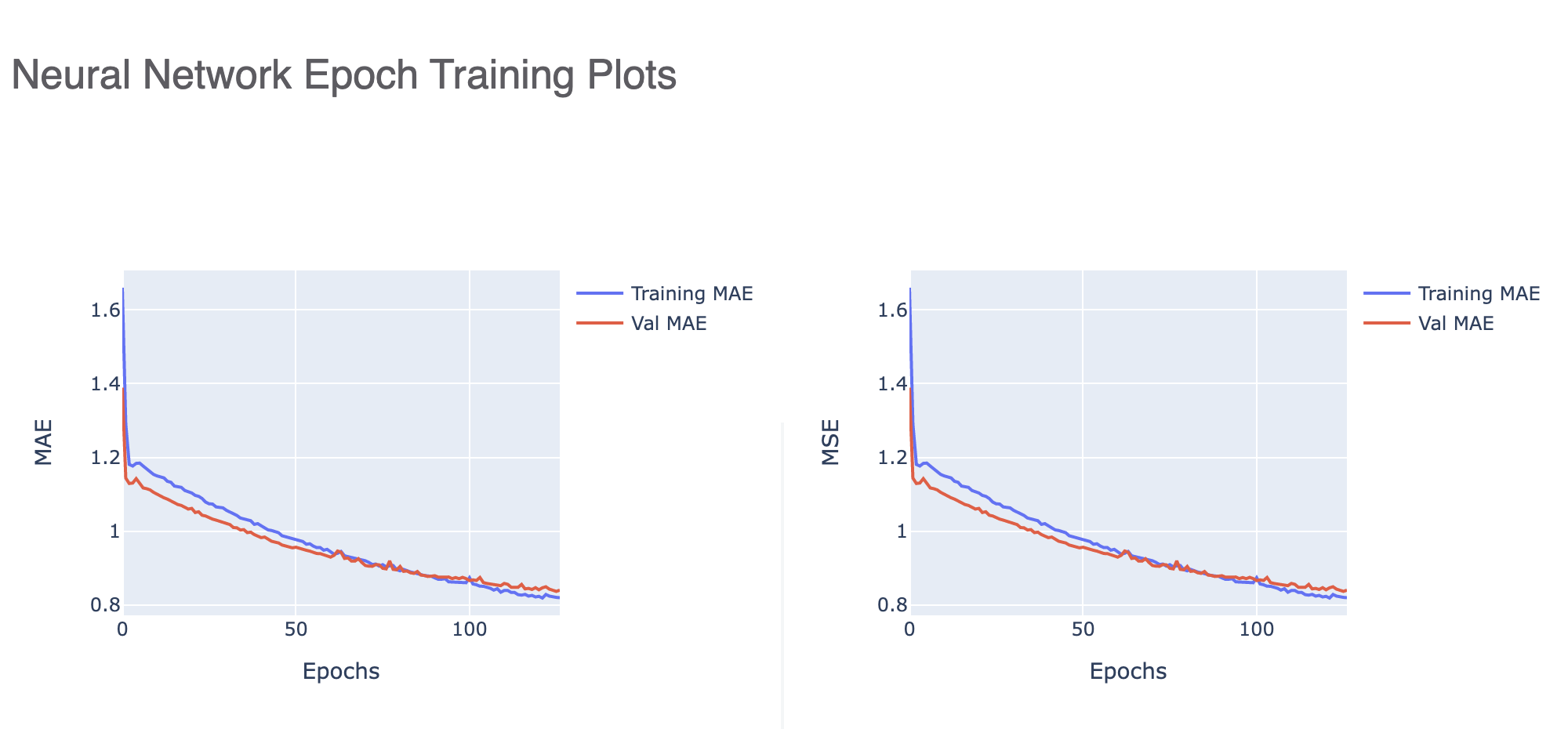# How-to: Build an Optimal Property Predicting Machine Learning Model by Tweaking Neural Network Architecture¶

OpenEye Machine Learning builds machine learning models that predict properties of small molecules.

You can can build multiple learning models based on a set of different Cheminformatics and machine-learning hyperparameters. The new Keras Hyperparamter tuner optimizes these hyperparameters to further fine tune built models. The floe report gives you a chance to analyze how well the models are trained and pick the best for future property prediction on unseen molecules.

The Model Building floe generates several neural network models for every different combination of parameter values supplied. Refer to tutorial Building Machine Learning Regression Models on how they are built. Thus, if there are n parameters [p1,..pn], with p1 having v1 different values, p2 having v2 different values and so on, there will be [v1*v2*..*vn]*k models built. The ‘k’ value refers to the additional models the Keras tuner builds for each hyperparameter combination supplied. This approach is inspired from the commonly used Grid Search Technique ( Grid Search Introduction ) in the Neural Network community. On top of every course grained grid search, we further fine tune the model building process by using tuners as RandomSearch, Hyperband and Bayesian Optimization. You can take the help of the floe report to pick the best model to predict properties of previously unseen/new molecules.

• Build models using the following Regression Floe:

• Floe name: ML Build: Regression Model with Tuner using Fingerprints for Small Molecules

• Model Learns and predicts Exact Values. Examples may include:

• hERG Toxicity Level in IC50

• Permeability Level in IC50 concentration

• Solubility Level mol/L

• Floe name: ML Build: Regression Model with Tuner using Fingerprints for Small Molecules
• To run probability models, turn ON Parameter: Are We Training Tensorflow Probability

• This options Builds TensorFlow Probability Models

• TFP models are Better at predicting error bars/ Domain of Application

• Refer to tutorial Predict Generic Property of Molecules to see how these models can be put to action

• Note: this option does not use the new Keras Hyperparameter Tuner

This tutorial uses the following Floe: ML Build: Regression Model with Tuner using Fingerprints for Small Molecules

For each run of the Model Build tool, several Fully Connected Feed Forward Neural Networks (Tutorial FCNN) are built which predict physical properties of molecules.

## Fully Connected Feed Forward Neural Network (Fnn)¶

The definition of Artificial Neural Network as provided by the inventor of one of the first neurocomputers, Dr. Robert Hecht-Nielsen:

” ..a computing system made up of a number of simple, highly interconnected processing elements, which process information by their dynamic state response to external inputs.” Simply put, neural network is a set of interconnected nodes or mathematical modules (just like neurons in our brain) which collectively tries to learn a phenomenon (like how permeability is related to a molecule’s composition). It does so by iteratively looking at the training examples (molecular fingerprints for our case) and predicting the property we wish to learn.

Architecture

The following figures show the architecture of a neural network (left) and a single node (right)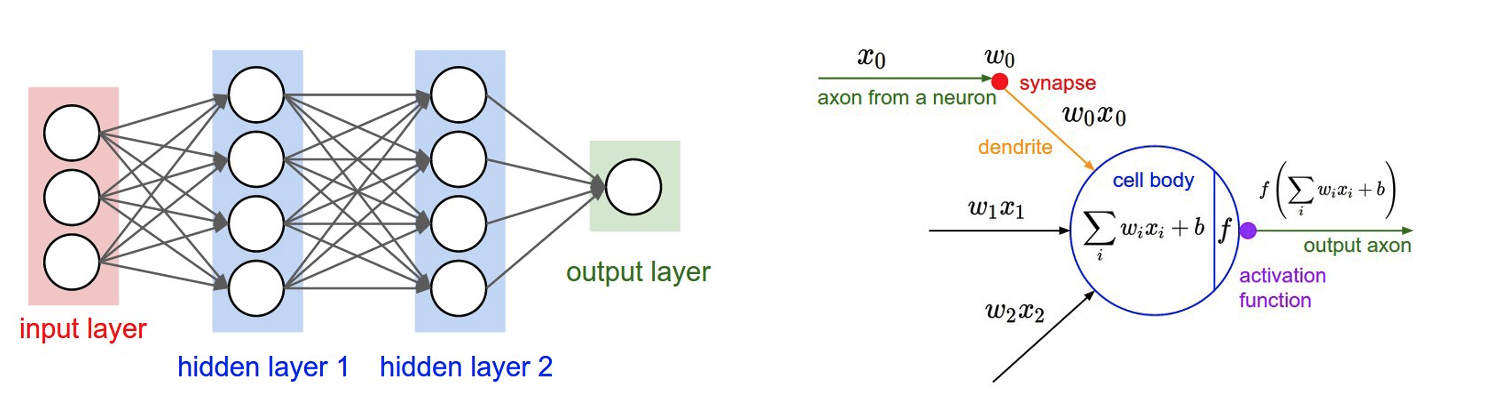The Fnn need a feature vector to train the network on. As we see from the figure, the size of the first layer is the same size as our input feature vector. We convert small molecules to a feature vector by leveraging Cheminformatics based fingerprints (Molecule OE Fingerprint). Users can specify the type (p1), bit length (p2), max radius (p3), and min radius (p4) of these fingerprints in the Cheminformatics Fingerprints Option. Thus v1 maybe Circular, Tree, or Path; v2 may be 2048, 4096 etc. When choosing p2, you should be mindful of the relation between feature vector and the size of the input dataset. One such emperical relation is stated in Hua et al :

• For uncorrelated features, the optimal feature size is N−1 (where N is sample size).

• As feature correlation increases, the optimal feature size becomes proportional to √N for highly correlated features.

This is Optimization Strategy I, setting bit length in accordance to training size.

We can also choose our cheminformatics features based on nature of the physical property we are trying to predict. For instance, as solubility is more of a local fragment property, keeping min and max radius of the fingerprint on the lower end would yeild better results. In constrast, properties where different fragments of the molecule interact more may benefit from larger radius sizes. [Optimization Strategy II]

Besides the cheminfo features, we may also tweak the machine learning hyperparameters available as the Neural Network Training Hyperparameter Options.

Note that the parameters takes in list of values and models will be trained for every possible combination with the other parameters as stated above. Among these parameters, the Sets of Hidden Layters is of particular interest as it dictates the number of nodes in each layer of the network.

These hidden nodes allow the network to learn and exhibit non-linear behavior.

Thus, if the total number of nodes is too low, it might be insufficient for the network to learn the prediction function. However, large number of hidden nodes makes the network learn the training set very minutely, thereby loosing its ability to generalise. In this case, it would perform poorly for unseen samples. This conundrum of too small, versus too large hidden layer is known as the underfitting and overfittting problem. A simple rule of thumb to determine this is [Optimization Strategy III]:

Nh = Ns/(a*(Ni+No))

• Ni = number of input neurons. (Equals bit length p2)

• No = number of output neurons. (1 for regression networks, >=2 for classification networks)

• Ns = number of samples in training data set.

• a = an arbitrary scaling factor usually 2-10.

In addition, parameters as dropouts and regularisers help with overfitting as well. Users can train on a set of dropouts, and can set a value for L2 regularisers in the Neural Network Hyperparameter Training Option.

Dropouts act by randomly turning off a certain percentage of the node while training. This introduces non-deterministicity in the model there generalizing it for unseen data.

Regularisers are a great way to prevent overfitting, ie the model learning too much details on the training set that is it unable to predict for unseen molecules. While there are many techniques to regulariser, we choose R2 regularisers since they are smoother and converge easily. Here is an example of a training history of a model which suggest divergence. Adding reguliser would certainly improve the ability of the model to generalise.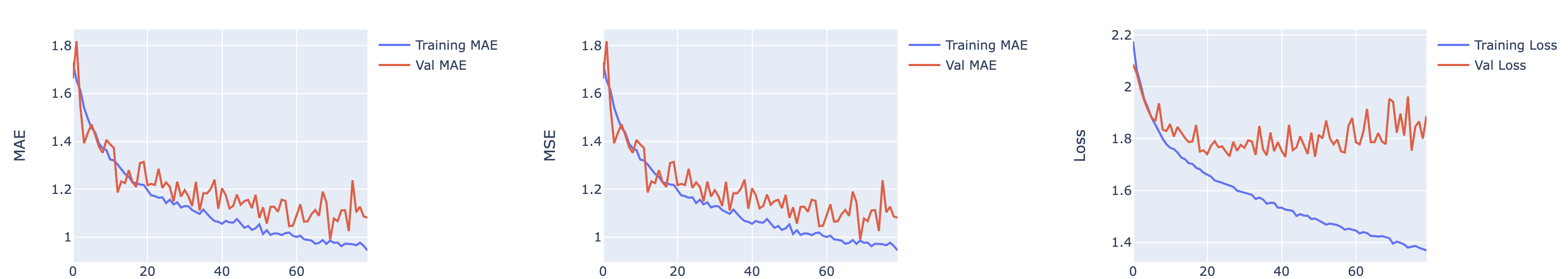Once a model is trained, we can look into the training graphs in the Floe-report (Model Link) to gather more insight into the quality of the model built. The graphs illustrate how the mean absolute error (MAE) and mean squared error (MSE) change with every epoch of training. The first picture tells us that the learning rate is too high leading to large fluctuations in every epoch.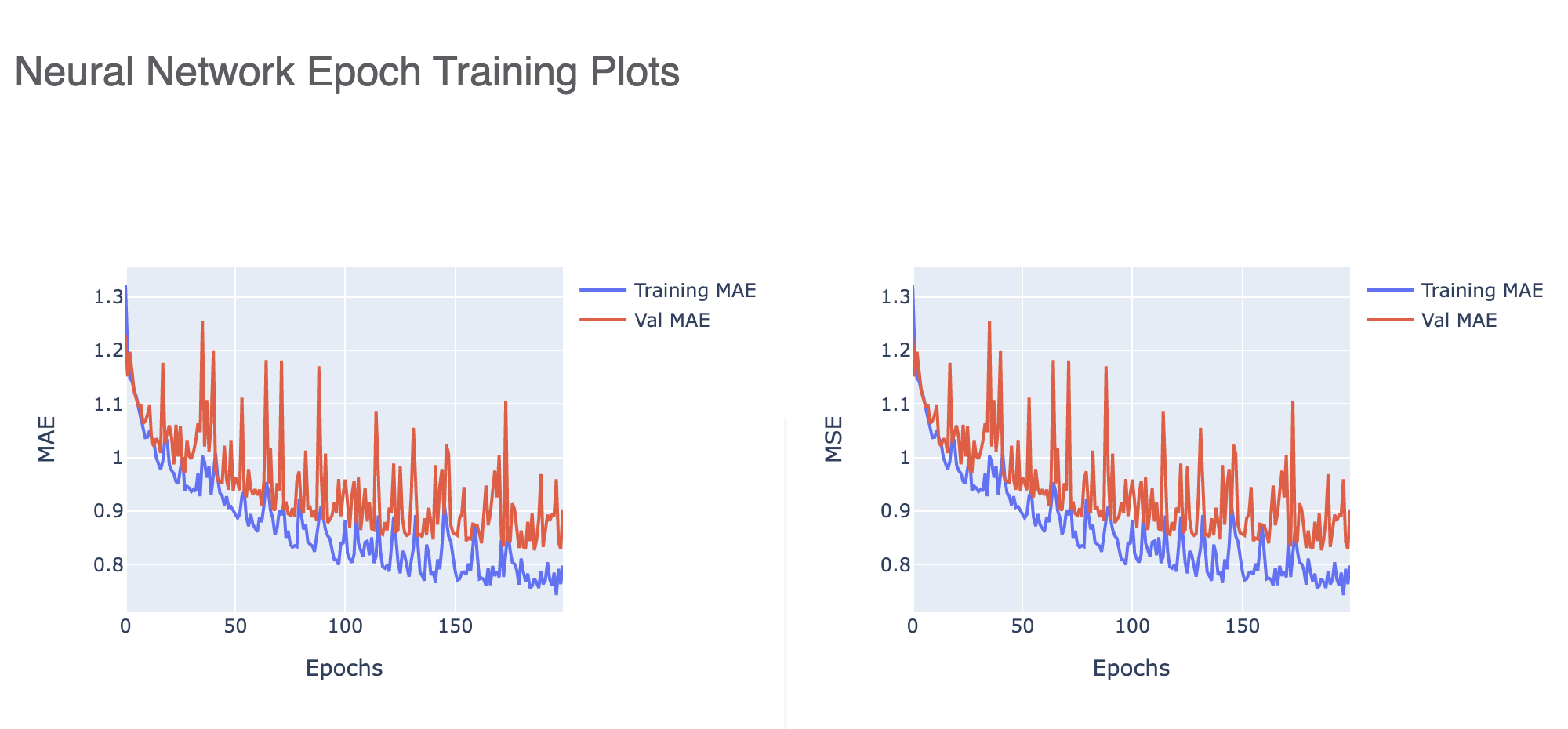This one tells us that the validation error is much higher than the training meaning we have overfit our model. Increasing the regularisation parameter or decreasing the number of nodes might be a good way to stop overfitting [Optimization Strategy IV].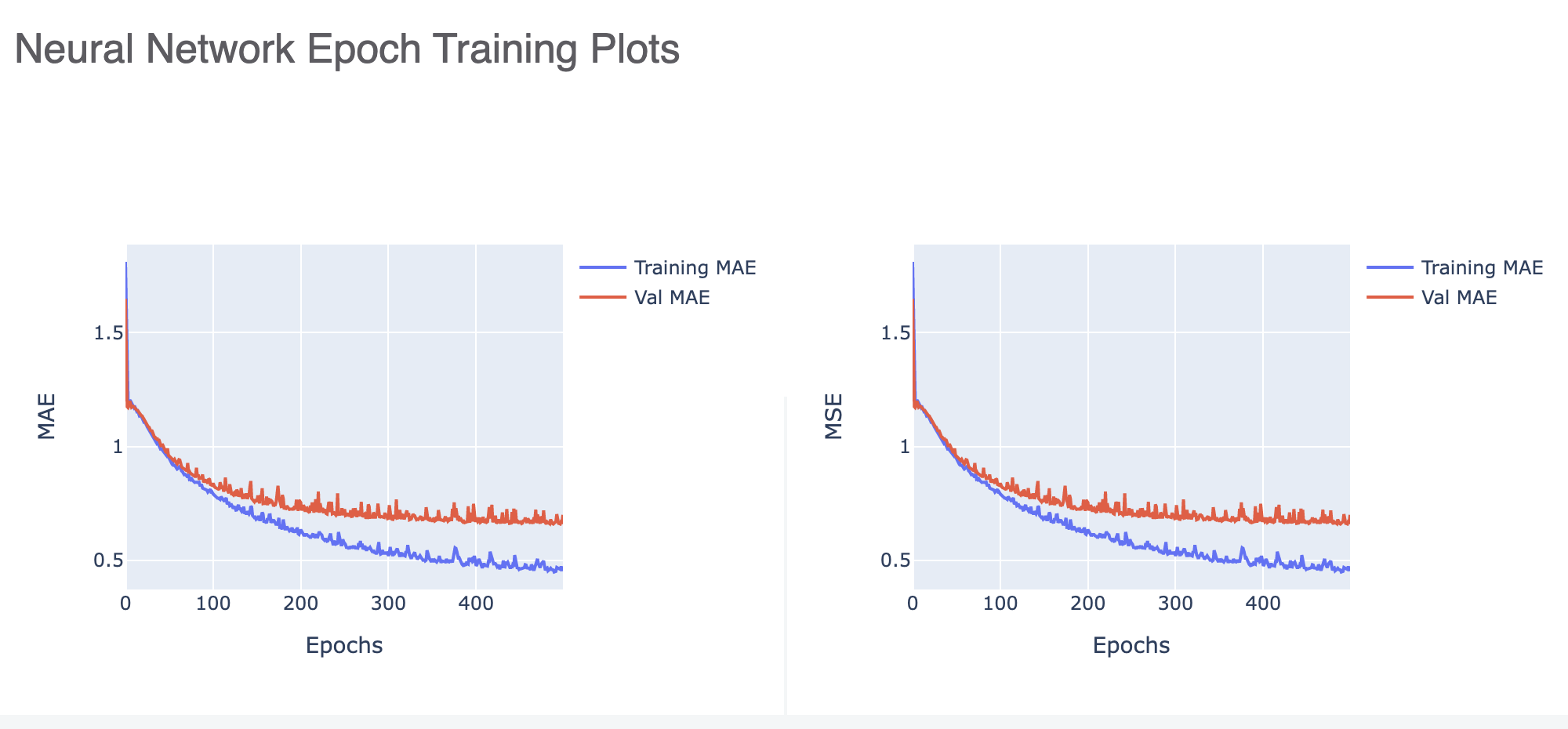Finally this picture shows us how a well trained model should look like.